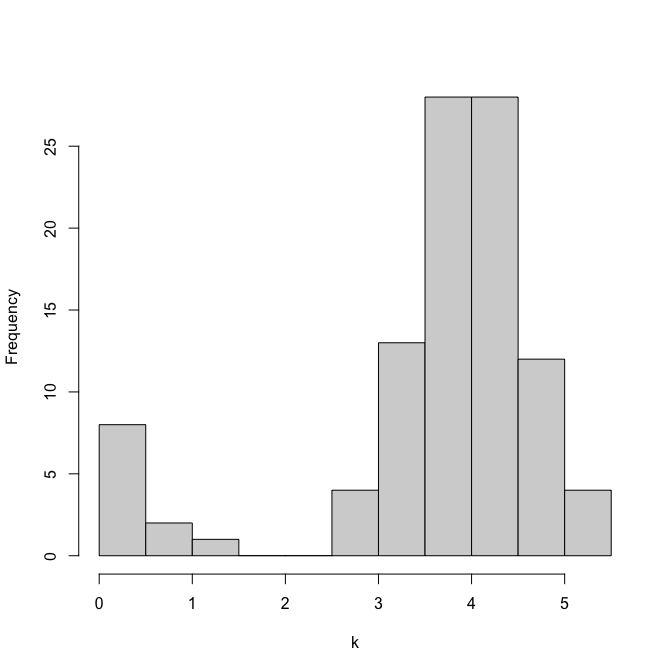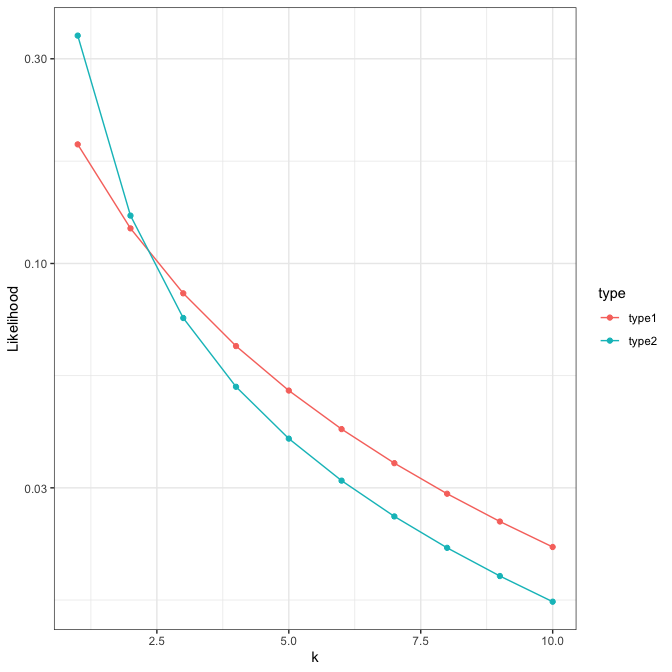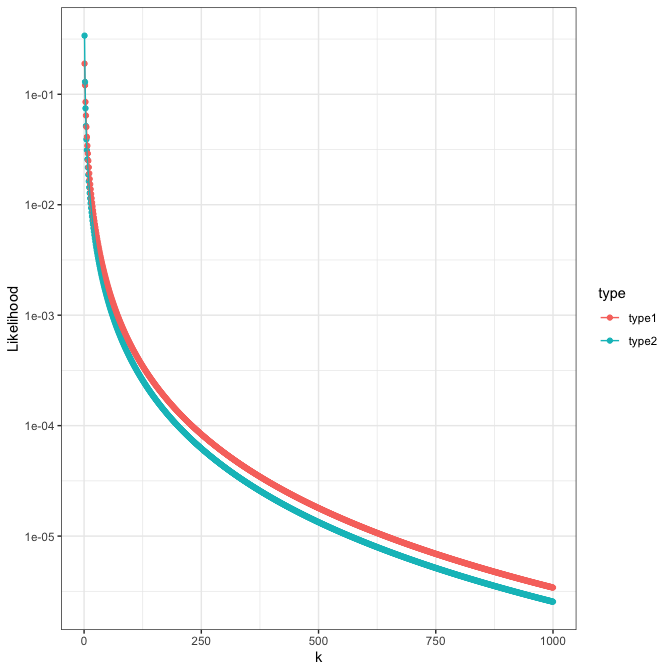# Zero Truncated Poisson Lognormal Distribution

A compound Poisson-lognormal distribution (PLN) is a Poisson probability distribution where its parameter $$\lambda$$ is a random variable with lognormal distribution, that is to say $$log \lambda$$ are normally distributed with mean $$\mu$$ and variance $$\sigma^2$$ (Bulmer 1974). The density function is

$\mathcal{PLN} (k ; \mu, \sigma) = \int_0^\infty {Pois}(k; \lambda) \times \mathcal{N}(log\lambda; \mu, \sigma) d\lambda \\ = \frac{1}{\sqrt{2\pi\sigma^2}k!}\int^\infty_0\lambda^{k}exp(-\lambda)exp(\frac{-(log\lambda-\mu)^2}{2\sigma^2})d\lambda, \; \text{where} \; k = 0, 1, 2, ... \; \;\;(1).$

## ZTPLN - type 1

The zero-truncated Poisson-lognormal distribution (ZTPLN) at least have two different forms. First, it can be derived from a Poisson-lognormal distribution,

$\mathcal{PLN}_{zt}(k ; \mu, \sigma) = \frac{\mathcal{PLN}(k ; \mu, \sigma)}{1-\mathcal{PLN}(0 ; \mu, \sigma)}, \; \text{where} \; k = 1, 2, 3, ... \;\;(2).$

Random samples from ZTPLN (eq 2.) requires the inverse cumulative distribution function of ZTPLN (eq 2.),

$G_{zt}(x; \lambda) =\sum_{i=1}^k \mathcal{PLN}_{zt}(i ; \mu, \sigma) \;\;(3).$

Random sampling using $$G_{zt}^{-1}$$ is not implemented at the moment. Instead, zero values will be discarded from random variates that are generated by the inverse cumulative distribution of PLN.

## ZTPLN - type 2

An alternative form of ZTPLN can be directly derived from a mixture of zero-truncated Poisson distribution and lognormal distribution,

$\mathcal{PLN}_{zt2} (k ; \mu, \sigma) = \int_0^\infty \frac{{Pois}(k; \lambda)}{1 - Pois(0; \lambda)} \times \mathcal{N}(log\lambda; \mu, \sigma) d\lambda \\ = \frac{1}{\sqrt{2\pi\sigma^2}k!}\int^\infty_0 \frac{\lambda^{k}}{exp(\lambda) - 1}exp(\frac{-(log\lambda-\mu)^2}{2\sigma^2})d\lambda, \; \text{where} \; k = 1, 2, 3,... \; \;\;(4).$

Random samples from ZTPLN (eq. 3) can be generated by inverse transform sampling with the cumulative distribution function of a zero-truncated Poisson distribution ($$F_{zt}$$),

$F_{zt2}^{-1}(x; \lambda) = F^{-1}((1 - Pois(0; \lambda)) x + Pois(0; \lambda); \lambda) \\ = F^{-1}((1 - e^{-\lambda}) x + e^{-\lambda}; \lambda) \;\;\;\;(5)$

where $$F^{-1}_{zt2}$$ and $$F^{-1}$$ are inverse cumulative distribution function for a zero-truncated Poisson distribution and a Poisson distribution, respectively.

## Mixture models

A Poisson lognormal ($$\mathcal{PLN}$$) mixture model with K components for variables $$\boldsymbol y = (y_1, \ldots, y_N)$$ is parameterized by the mixture component weight vector $$\boldsymbol \theta = (\theta_1, \ldots, \theta_K)$$ such that $$0 \le \theta_{k} \le 1$$ and $$\sum\theta_{k} = 1$$, and the mean $$\boldsymbol \mu = (\mu_1, \ldots, \mu_K)$$ and standard deviation $$\boldsymbol \sigma = (\sigma_1, \ldots, \sigma_K)$$ of the variable’s natural logarithm. The probability mass function ($$p$$) for a Poisson lognormal mixture is

$p(\boldsymbol y; {\boldsymbol \mu}, {\boldsymbol \sigma}, {\boldsymbol \theta}) = \sum_{k=1}^{K}\theta_k \mathcal{PLN}(\boldsymbol{y} ; \mu_k, \sigma_k) \; \; (6).$

Given that each mixture component is zero-truncated, the probability mass function for the zero-truncated Poisson lognormal mixture ($$p_{zt}$$) is

$p_{zt}(\boldsymbol y; {\boldsymbol \mu}, {\boldsymbol \sigma}, {\boldsymbol \theta}) = \sum_{k=1}^{K}\theta_k \frac{\mathcal{PLN}(\boldsymbol{y} ; \mu_k, \sigma_k)} {1 - \mathcal{PLN}(0 ; \mu_k, \sigma_k)} \; \; (7)$

for ZTPLN type 1, and

$p_{zt2}(\boldsymbol y; {\boldsymbol \mu}, {\boldsymbol \sigma}, {\boldsymbol \theta}) = \sum_{k=1}^{K}\theta_k \mathcal{PLN}_{zt2}(\boldsymbol{y} ; \mu_k, \sigma_k) \; \; (8)$

for ZTPLN type 2.

## Functions

• dztpln(x, mu, sig, log = FALSE, type1 = TRUE): gives the (log) density of a zero truncated poisson lognormal distribution
• rztpln(n, mu, sig, type1 = TRUE): random draw from a zero truncated poisson lognormal distribution
• dztplnm(x, mu, sig, theta, log = FALSE, type1 = TRUE): gives the (log) density of a zero truncated poisson lognormal distribution mixture.
• ztplnm(n, mu, sig, theta, type1 = TRUE): random draw from a zero truncated poisson lognormal distribution mixture.

## Examples

### Random variates

We can generate ZTPLN random variates.

library(dplyr)
#>
#> Attaching package: 'dplyr'
#> The following objects are masked from 'package:stats':
#>
#>     filter, lag
#> The following objects are masked from 'package:base':
#>
#>     intersect, setdiff, setequal, union
library(tidyr)
library(ggplot2)
set.seed(123)

rztpln(n = 10, mu = 0, sig = 1)
#>   1 1 1 1 2 6 1 7 2 1

rztpln(n = 10, mu = 6, sig = 4)
#>      20   155     5    24   208   714  6221  2207    36 13624

We can also generate mixture of ZTPLN random variates.

rztplnm(n = 100,
mu = c(0, 4),
sig = c(0.5, 0.5),
theta = c(0.2, 0.8)) %>%
log %>% hist(main = "", xlab = "k")### Maximum likelihood estimation

Type 1 model (dztpln(..., type1 = TRUE)) fits better for type 1 random variates (rztpln(..., type1 = TRUE)).

y <- rztpln(n = 100, mu = 2, sig = 5, type1 = TRUE)
sum(dztpln(y, mu = 2, sig = 5, log = TRUE, type1 = TRUE))
#>  -764.0654
sum(dztpln(y, mu = 2, sig = 5, log = TRUE, type1 = FALSE))
#>  -790.5514

Type 2 model (dztpln(..., type1 = FALSE)) fits better for type 2 random variates (rztpln(..., type1 = FALSE)).

y2 <- rztpln(n = 100, mu = 2, sig = 5, type1 = FALSE)
sum(dztpln(y2, mu = 2, sig = 5, log = TRUE, type1 = TRUE))
#>  -571.5711
sum(dztpln(y2, mu = 2, sig = 5, log = TRUE, type1 = FALSE))
#>  -545.3733

### Probability density plots

Let’s consider a simple example where random variates follows the same parameters of type 1 and type 2 ZTPLN. We define two different sets of random variates, $$\mathcal{PLN}_{zt}(k ;1, 2)$$ and $$\mathcal{PLN}_{zt2}(k ; 1, 2)$$.

In this example, when $$k$$ is small, type 2 ZTPLN shows greater likelihood.

k1 <- 1
k <- 1000
dat <- tibble(type1 = dztpln(k1:k, mu = 1, sig = 2, type1 = TRUE),
type2 = dztpln(k1:k, mu = 1, sig = 2, type1 = FALSE),
x = k1:k) %>%
pivot_longer(-x, names_to = "type", values_to = "y")

ggplot(dat %>% dplyr::filter(x <= 10), aes(x = x, y = y, col = type)) +
geom_point() +
geom_line() +
scale_y_log10() +
xlab("k") +
ylab("Likelihood") +
theme_bw()When $$k$$ is large, type 1 ZTPLN shows greater likelihood.

ggplot(dat, aes(x = x, y = y, col = type)) +
geom_point() +
geom_line() +
scale_y_log10() +
xlab("k") +
ylab("Likelihood") +
theme_bw()## Reference

Bulmer, M. G. 1974. On Fitting the Poisson Lognormal Distribution to Species-Abundance Data. Biometrics 30:101-110.

Inouye, D., E. Yang, G. Allen, and P. Ravikumar. 2017. A Review of Multivariate Distributions for Count Data Derived from the Poisson Distribution. Wiley interdisciplinary reviews. Computational statistics 9:e1398.